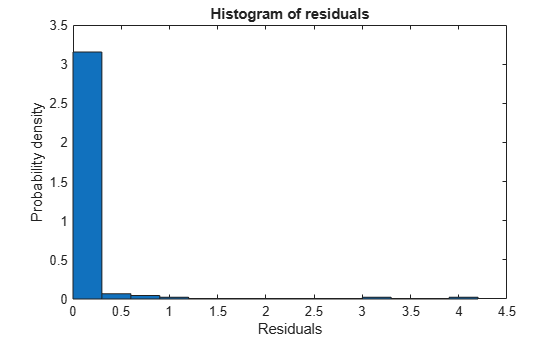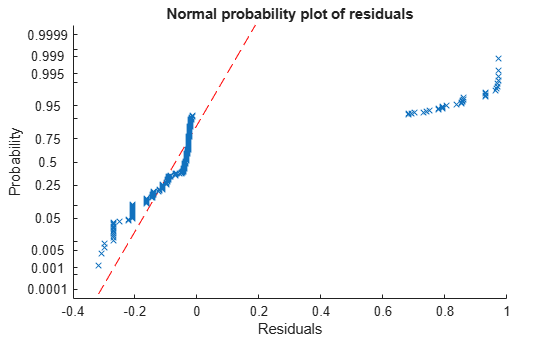# plotResiduals

Plot residuals of multinomial regression model

Since R2023a

## Syntax

``plotResiduals(mdl)``
``plotResiduals(mdl,plotType)``
``plotResiduals(ax,___)``
``plotResiduals(___,Name=Value)``
``h = plotResiduals(___)``

## Description

example

````plotResiduals(mdl)` generates a probability density plot of the deviance residuals for the multinomial regression model object `mdl`.```

example

````plotResiduals(mdl,plotType)` specifies the residual plot type.```
````plotResiduals(ax,___)` plots on the axes specified by `ax` instead of the current axes, using any of the input argument combinations in previous syntaxes.```
````plotResiduals(___,Name=Value)` specifies additional options using one or more name-value arguments. For example, you can specify the type of residuals to plot and the colors of the plotted objects.```

example

````h = plotResiduals(___)` returns graphics objects for the lines or patch in the plot. Use `h` to modify the properties of a specific line or patch after you create the plot. For a list of properties, see Line Properties or Patch Properties.```

## Examples

collapse all

Load the `fisheriris` sample data set.

`load fisheriris`

The column vector `species` contains three iris flower species: setosa, versicolor, and virginica. The matrix `meas` contains four types of measurements for the flowers: the length and width of sepals and petals in centimeters.

Fit a multinomial regression model to predict the iris flower species using the measurements. Display the table of residuals for the fitted model.

```mdl = fitmnr(meas,species); mdl.Residuals```
```ans=150×3 table Raw Pearson Deviance ___________ ___________ ________ 0 0 0 0 0 0 0 0 0 0 0 0 0 0 0 0 0 0 0 0 0 0 0 0 0 0 0 0 0 0 0 0 0 0 0 0 0 0 0 0 0 0 0 0 0 0 0 0 0 0 0 0 0 0 0 0 0 0 0 0 0 0 0 0 0 0 0 0 0 0 0 0 0 0 0 0 0 0 0 0 0 0 0 0 0 0 0 0 0 0 0 0 0 0 0 0 0 0 0 0 0 0 0 0 0 0 0 0 0 0 0 0 ⋮ ```

The output shows the raw, Pearson, and deviance residuals for the model.

Generate a probability density plot of the deviance residuals.

`plotResiduals(mdl)`The histogram shows that most of the deviance residuals are small. However, several residuals are greater than one.

Inspect the observations with larger residuals. Sort the residuals from largest to smallest by using the `sort` function. Use the `head` function to display the four largest residuals and their corresponding indices.

```[r, ind] = sort(mdl.Residuals.Deviance,"descend"); head(r,4)```
``` 4.0443 3.1707 1.0378 0.8035 ```
`head(ind,4)`
``` 84 134 71 139 ```

The outputs show that observations corresponding to rows 84, 134, and 71 of `meas` and `species` have residuals larger than one. Given that most other residuals are close to zero, observations 84, 134, and 71 are most likely outliers.

Load the `carbig` sample data set.

`load carbig`

The vectors `Displacement`, `Cylinders`, and `Model_Year` contain data for car engine displacement, number of engine cylinders, and year the car was manufactured, respectively.

Fit a multinomial regression model using `Displacement` and `Cylinders` as predictors variables and `Model_Year` as the response.

`mdl = fitmnr([Displacement Cylinders],Model_Year);`

To determine whether the residuals are random, generate a lagged plot of the deviance residuals.

`plotResiduals(mdl,"lagged")`The lagged plot of the deviance residuals shows a cluster of residuals trending toward the top right of the plot. The cluster indicates a mildly positive correlation between the residual for a data point and the residual for the previous data point, suggesting that the residuals might not be random.

Load the `carbig` sample data set.

`load carbig`

The vectors `Displacement`, `Cylinders`, and `Model_Year` contain of data for car engine displacement, number of engine cylinders, and year the car was manufactured, respectively.

Fit a nominal multinomial regression model using `Displacement` and `Cylinders` as predictors variables and `Model_Year` as the response.

`mdl = fitmnr([Displacement Cylinders],Model_Year);`

`mdl` is a multinomial regression model object that contains the results of fitting a nominal multinomial regression model to the data.

Display the name of the first response category.

`mdl.ClassNames(1)`
```ans = 70 ```

The output shows that the first response category corresponds to cars manufactured in 1970.

To determine whether the raw residuals for cars manufactured in 1970 are randomly distributed, create a probability plot and return a graphics array of the plotted objects. By default, `plotResiduals` uses the raw residuals for the first response category to create the probability plot.

`h = plotResiduals(mdl,"probability",ResidualType="raw")`
```h = 2×1 graphics array: Line (main) FunctionLine ```

The output shows the data types for the elements in the graphics array `h`. The `Line` element corresponds to the raw residuals. The `FunctionLine` element corresponds to the line representing the theoretical normal distribution.

Specify the color red for the normal distribution line by modifying the `Color` property of the second element in `h`.

`h(2).Color = "r"````h = 2×1 graphics array: Line (main) FunctionLine ```

The plot shows that the residuals do not follow the red line, indicating that the raw residuals for cars manufactured in 1970 are not normally distributed.

## Input Arguments

collapse all

Multinomial regression model object, specified as a `MultinomialRegression` model object created with the `fitmnr` function.

Plot type, specified as one of the following values.

ValueDescription
`"histogram"`Probability density plot for residuals (default)
`"caseorder"`Residuals versus case (row) order
`"lagged"`Residuals versus lagged residuals—that is, r(i) versus r(i – 1), where r(i) is the residual for the ith data point
`"probability"`Normal probability plot
`"symmetry"` Symmetry plot

Example: `"lagged"`

Data Types: `char` | `string`

Target axes, specified as an `Axes` object. If you do not specify the axes, then `plotResiduals` uses the current axes (`gca`).

### Name-Value Arguments

Specify optional pairs of arguments as `Name1=Value1,...,NameN=ValueN`, where `Name` is the argument name and `Value` is the corresponding value. Name-value arguments must appear after other arguments, but the order of the pairs does not matter.

Example: `plotResiduals(mdl,ResidualType="Pearson",ClassToPlot="virginica",FaceColor="m")` generates a magenta histogram for the Pearson residuals of the `virginica` response category.

Residuals

collapse all

Type of residual to plot, specified as one of the options in the following table.

OptionDescription
`"deviance"`Deviance residuals (default)
`"raw"`

Raw residuals, which are the observed values minus the fitted values

`"Pearson"`

Pearson residuals, which are the raw residuals divided by the root mean squared error (RMSE)

Example: `ResidualType="raw"`

Data Types: `char` | `string`

Residual response category to plot, specified as the name of a response category used to fit `mdl`. You can view the list of response category names by using the property `mdl.ClassNames`. The default value of `ClassToPlot` is `mdl.ClassNames(1)`. This argument is valid only when `ResidualType` is `"Pearson"` or `"raw"`.

Example: `ClassToPlot="virginica"`

Data Types: `single` | `double` | `logical` | `char` | `cell` | `categorical`

Histogram Graphics

collapse all

Face color, specified as `"interp"`, `"flat"` an RGB triplet, a hexadecimal color code, a color name, or a short name.

To designate a single color for all faces, specify `FaceColor` as an RGB triplet, a hexadecimal color code, a color name, or a short name.

• An RGB triplet is a three-element row vector whose elements specify the intensities of the red, green, and blue components of the color. The intensities must be in the range `[0,1]`; for example, `[0.4 0.6 0.7]`.

• A hexadecimal color code is a character vector or a string scalar that starts with a hash symbol (`#`) followed by three or six hexadecimal digits, which can range from `0` to `F`. The values are not case sensitive. Thus, the color codes `"#FF8800"`, `"#ff8800"`, `"#F80"`, and `"#f80"` are equivalent.

Color NameShort NameRGB TripletHexadecimal Color CodeAppearance
`"red"``"r"``[1 0 0]``"#FF0000"``"green"``"g"``[0 1 0]``"#00FF00"``"blue"``"b"``[0 0 1]``"#0000FF"``"cyan"` `"c"``[0 1 1]``"#00FFFF"``"magenta"``"m"``[1 0 1]``"#FF00FF"``"yellow"``"y"``[1 1 0]``"#FFFF00"``"black"``"k"``[0 0 0]``"#000000"``"white"``"w"``[1 1 1]``"#FFFFFF"``"none"`Not applicableNot applicableNot applicableNo color

Here are the RGB triplets and hexadecimal color codes for the default colors MATLAB® uses in many types of plots.

`[0 0.4470 0.7410]``"#0072BD"``[0.8500 0.3250 0.0980]``"#D95319"``[0.9290 0.6940 0.1250]``"#EDB120"``[0.4940 0.1840 0.5560]``"#7E2F8E"``[0.4660 0.6740 0.1880]``"#77AC30"``[0.3010 0.7450 0.9330]``"#4DBEEE"``[0.6350 0.0780 0.1840]``"#A2142F"`Example: `FaceColor="g"`

Data Types: `single` | `double` | `char` | `string`

Face transparency, specified as one of these values:

• Scalar in the range `[0,1]` — Use uniform transparency across all of the faces. A value of `1` is fully opaque and `0` is completely transparent. This option does not use the transparency values in the `FaceVertexAlphaData` property.

• `'flat'` — Use a different transparency for each face based on the values in the `FaceVertexAlphaData` property. First you must specify the `FaceVertexAlphaData` name-value argument as a vector containing one transparency value per face or vertex. The transparency value at the first vertex determines the transparency for the entire face.

• `'interp'` — Use interpolated transparency for each face based on the values in the `FaceVertexAlphaData` property. First you must specify the `FaceVertexAlphaData` name-value argument as a vector containing one transparency value per vertex. The transparency varies across each face by interpolating the values at the vertices.

Example: `FaceAlpha=0.5`

Data Types: `single` | `double` | `char` | `string`

Line width, specified as a positive value in points, where 1 point = 1/72 of an inch. If the line has markers, then the line width also affects the marker edges.

The line width cannot be thinner than the width of a pixel. If you set the line width to a value that is less than the width of a pixel on your system, the line appears as one pixel wide.

Example: `LineWidth=1.5`

Data Types: `single` | `double`

Note

The graphics properties for histograms listed here are only a subset. For a complete list, see Patch Properties.

Line Graphics

collapse all

Line color, specified as an RGB triplet, a hexadecimal color code, a color name, or a short name. The default value of `[0 0 0]` corresponds to black.

For a custom color, specify an RGB triplet or a hexadecimal color code.

• An RGB triplet is a three-element row vector whose elements specify the intensities of the red, green, and blue components of the color. The intensities must be in the range `[0,1]`, for example, `[0.4 0.6 0.7]`.

• A hexadecimal color code is a character vector or a string scalar that starts with a hash symbol (`#`) followed by three or six hexadecimal digits, which can range from `0` to `F`. The values are not case sensitive. Therefore, the color codes `"#FF8800"`, `"#ff8800"`, `"#F80"`, and `"#f80"` are equivalent.

Alternatively, you can specify some common colors by name. This table lists the named color options, the equivalent RGB triplets, and hexadecimal color codes.

Color NameShort NameRGB TripletHexadecimal Color CodeAppearance
`"red"``"r"``[1 0 0]``"#FF0000"``"green"``"g"``[0 1 0]``"#00FF00"``"blue"``"b"``[0 0 1]``"#0000FF"``"cyan"` `"c"``[0 1 1]``"#00FFFF"``"magenta"``"m"``[1 0 1]``"#FF00FF"``"yellow"``"y"``[1 1 0]``"#FFFF00"``"black"``"k"``[0 0 0]``"#000000"``"white"``"w"``[1 1 1]``"#FFFFFF"``"none"`Not applicableNot applicableNot applicableNo color

Here are the RGB triplets and hexadecimal color codes for the default colors MATLAB uses in many types of plots.

`[0 0.4470 0.7410]``"#0072BD"``[0.8500 0.3250 0.0980]``"#D95319"``[0.9290 0.6940 0.1250]``"#EDB120"``[0.4940 0.1840 0.5560]``"#7E2F8E"``[0.4660 0.6740 0.1880]``"#77AC30"``[0.3010 0.7450 0.9330]``"#4DBEEE"``[0.6350 0.0780 0.1840]``"#A2142F"`Example: `Color="blue"`

Example: `Color=[0 0 1]`

Example: `Color="#0000FF"`

Data Types: `single` | `double` | `char` | `string`

Line style, specified as one of the options listed in this table.

Line StyleDescriptionResulting Line
`"-"`Solid line`"--"`Dashed line`":"`Dotted line`"-."`Dash-dotted line`"none"`No lineNo line

Example: `LineStyle="--"`

Data Types: `char` | `string`

Marker symbol, specified as one of the values listed in this table. By default, the object does not display markers. Specifying a marker symbol adds markers at each data point or vertex.

MarkerDescriptionResulting Marker
`"o"`Circle`"+"`Plus sign`"*"`Asterisk`"."`Point`"x"`Cross`"_"`Horizontal line`"|"`Vertical line`"square"`Square`"diamond"`Diamond`"^"`Upward-pointing triangle`"v"`Downward-pointing triangle`">"`Right-pointing triangle`"<"`Left-pointing triangle`"pentagram"`Pentagram`"hexagram"`Hexagram`"none"`No markersNot applicable

Example: `Marker="x"`

Data Types: `char` | `string`

Note

The graphics properties for lines listed here are only a subset. For a complete list, see Line Properties.

## Output Arguments

collapse all

Handle to the plotted objects, returned as a `Patch` object or a graphics array.

When `plotType` is `"Histogram"`, `h` is a `Patch` object. Otherwise, `h` is a graphics array. The contents of the graphics array depend on the value of `plotType`.

`plotType Value`Contents of Graphics Array
`"caseorder"``[Line; Line]`
`"lagged"``[Line; Line; Line]`
`"probability"``[Line; FunctionLine]`
`"symmetry"``[Line; Line]`

## Version History

Introduced in R2023a